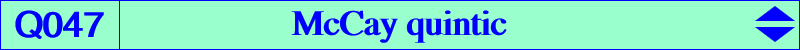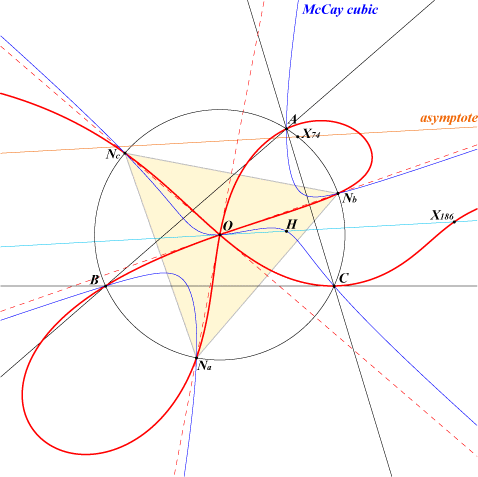too complicated to be written here. Click on the link to download a text file.X(3), X(30), X(36), X(186) Na, Nb, Nc : vertices of the circumnormal triangle inverses of the excenters in the circumcircle anti-points, see Table 77The locus of point P such that the circumcevian and reflection triangles of P are perspective is the McCay cubic. The locus of the perspector is Q047, the McCay quintic. Q047 is the inversive image of the McCay cubic in the circumcircle. It is a bicircular quintic with a real asymptote parallel at X(74) to the Euler line. O is a triple point on the curve with tangents the altitudes of the circumnormal triangle. K038 is the locus of points whose polar conic in Q047 is a rectangular hyperbola. In other words, the cubic is the Laplacian of the quintic.